## Tamilnadu Samacheer Kalvi 12th Maths Solutions Chapter 1 Applications of Matrices and Determinants Ex 1.8

Choose the correct or the most suitable answer from the given four alternatives.

Question 1.
If |adj(adj A)| = |A|9, then the order of the square matrix A is _______
(a) 3
(b) 4
(c) 2
(d) 5
(b) 4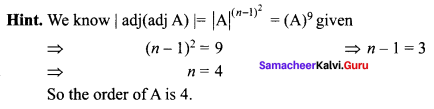Question 2.
If A is a 3 × 3 non-singular matrix such that AAT = ATA and B = A-1AT, then BBT = ______
(a) A
(b) B
(c) I3
(d) BT
(c) I3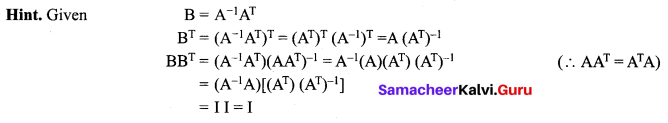Question 3.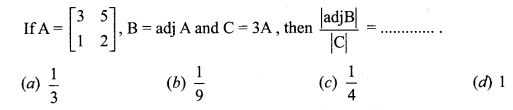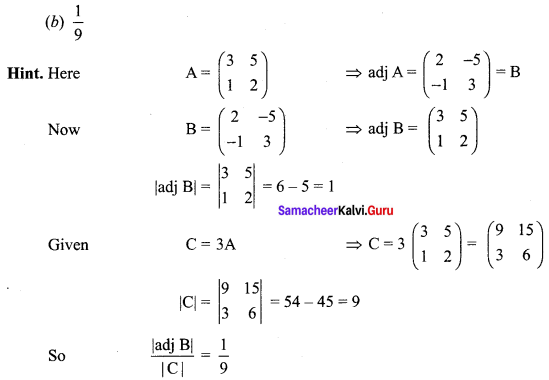Question 4.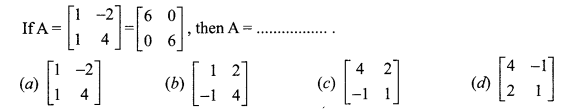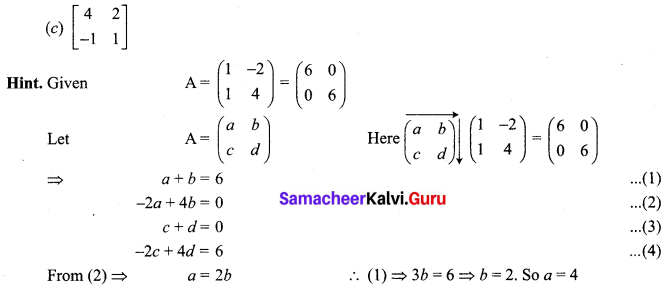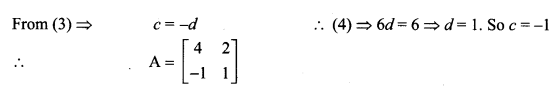Question 5.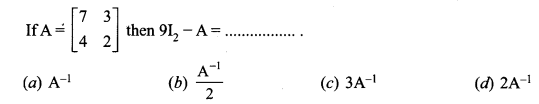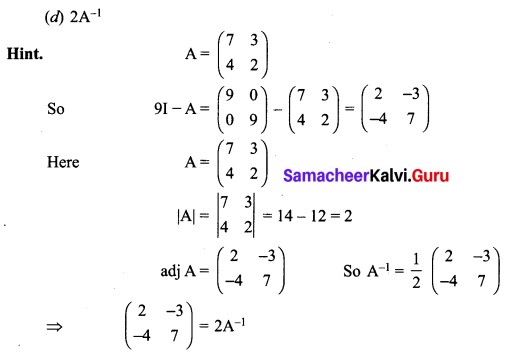Question 6.
If A = $$\left[\begin{array}{ll}{2} & {0} \\ {1} & {5}\end{array}\right]$$ and B = $$\left[\begin{array}{ll}{1} & {4} \\ {2} & {0}\end{array}\right]$$ then |adj(AB)| = _______
(a) -40
(b) -80
(c) -60
(d) -20
(b) -80
Hint: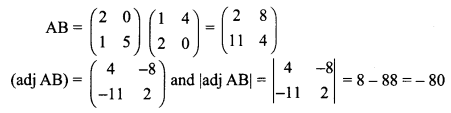Question 7.
If P = $$\left[\begin{array}{ccc}{1} & {x} & {0} \\ {1} & {3} & {0} \\ {2} & {4} & {-2}\end{array}\right]$$ is the adjoint of 3 × 3 matrix A and |A| = 4, then x is ______
(a) 15
(b) 12
(c) 14
(d) 11
(d) 11
Hint: Given |A| = 4 and P is the adjoint matrix of A
|P| = 42 = 16
⇒ -2 (3 – x) = 16
⇒ -6 + 2x = 16
⇒ 2x = 22
⇒ x = 11

Question 8.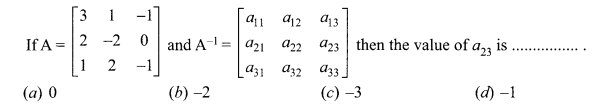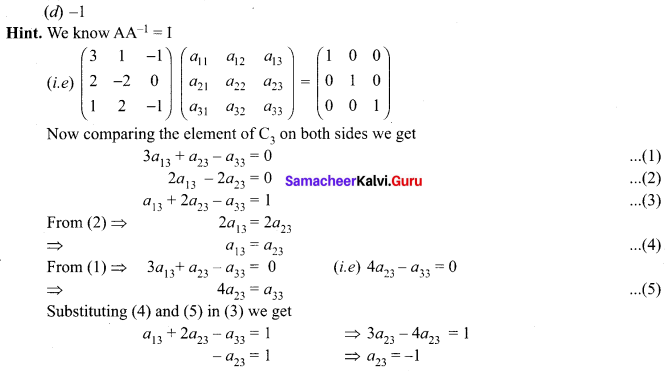Question 9.
If A, B and C are invertible matrices of some order, then which one of the following is not true?
(c) det A-1 = (det A)-1
(d) (ABC)-1 = C-1B-1A-1

Question 10.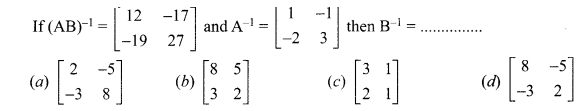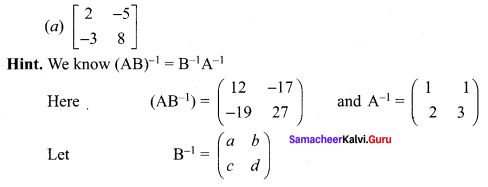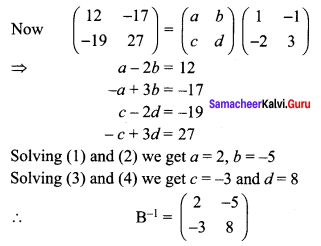Question 11.
If AT A-1 is symmetric, then A2 = _______
(a) A-1
(b) (AT)2
(c) AT
(d) (A-1)2
(b) (AT)2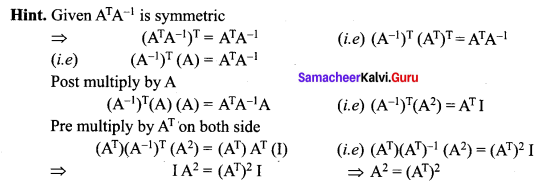Question 12.
If A is a non-singular matrix such that $$A^{-1}=\left[\begin{array}{rr}{5} & {3} \\ {-2} & {-1}\end{array}\right]$$, then (AT)-1 = _______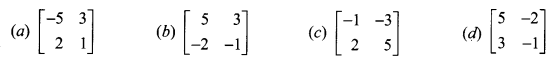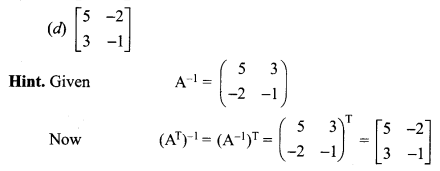Question 13.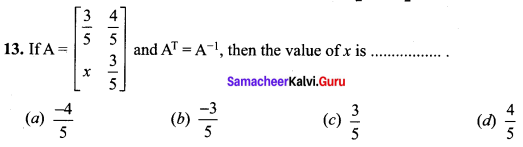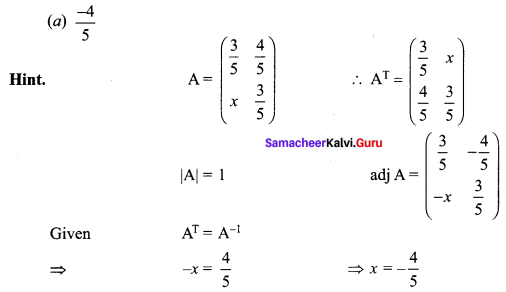Question 14.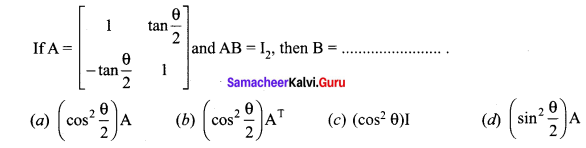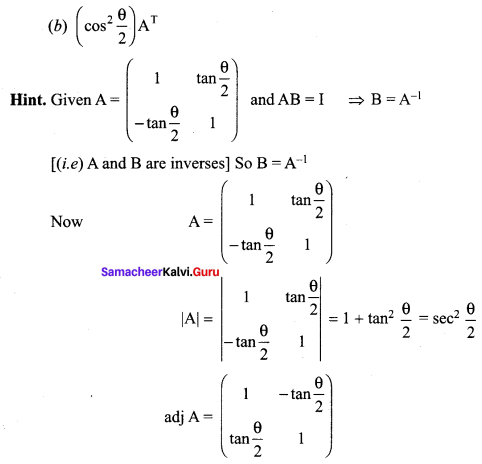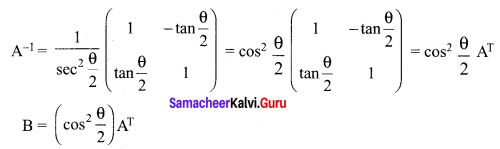Question 15.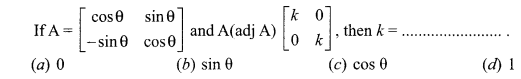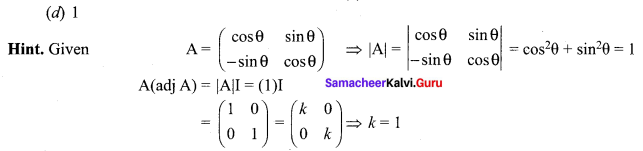Question 16.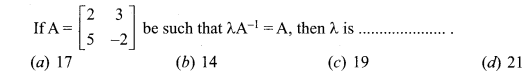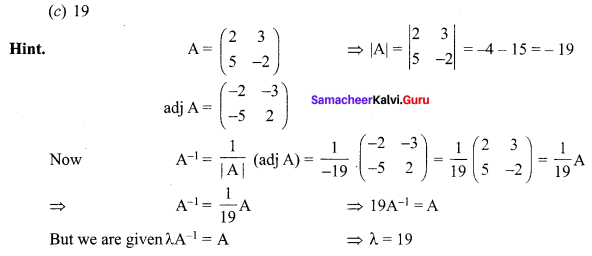Question 17.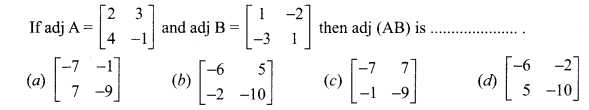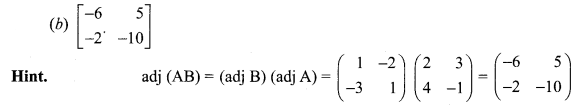Question 18.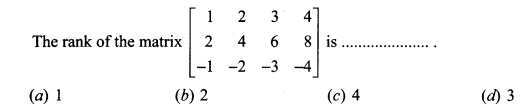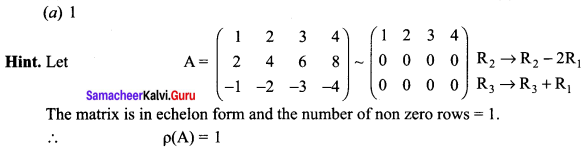Question 19.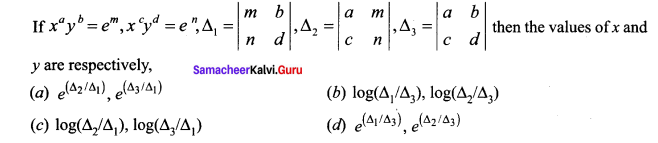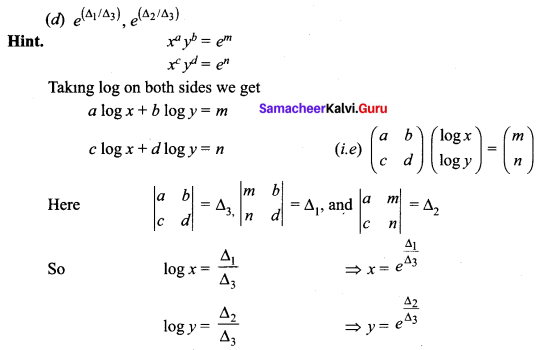Question 20.
Which of the following is/are correct?
(i) Adjoint of a symmetric matrix is also a symmetric matrix
(ii) Adjoint of a diagonal matrix is also a diagonal matrix.
(iii) If A is a square matrix of order n and λ, is a scalar, then adj(λA) = λn adj(A).
(a) Only (i)
(b) (ii) and (iii)
(c) (iii) and (iv)
(d) (i), (ii) and (iv)
(d) (i), (ii) and (iv)

Question 21.
If ρ(A) = ρ([A|B]), then the system AX = B of linear equations is ______
(a) consistent and has a unique solution
(b) consistent
(c) consistent and has infinitely many solution
(d) inconsistent
(b) consistent

Question 22.
If 0 ≤ θ ≤ π, the system of equations x + (sin θ)y – (cos θ)z = 0, (cos θ)x – y + z = 0, (sin θ)x + y – z = 0 has a non-trivial solution then θ is ______
(a) $$\frac{2 \pi}{3}$$
(b) $$\frac{3 \pi}{4}$$
(c) $$\frac{5 \pi}{4}$$
(d) $$\frac{\pi}{4}$$
(d) $$\frac{\pi}{4}$$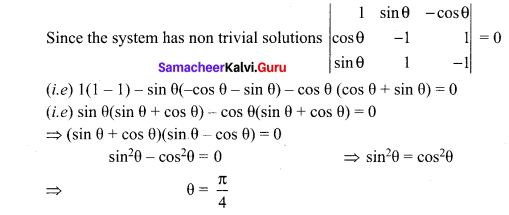Question 23.
The augmented matrix of a system of linear equations is $$\left[\begin{array}{cccc}{1} & {2} & {7} & {3} \\ {0} & {1} & {4} & {6} \\ {0} & {0} & {\lambda-7} & {\mu+5}\end{array}\right]$$. The system has infinitely many solutions if _____
(a) λ = 7, μ ≠ 5
(b) λ = -7, μ = 5
(c) λ ≠ 7, μ ≠ -5
(d) λ = 7, μ = -5
(d) λ = 7, μ = -5

Question 24.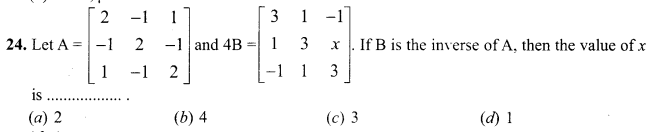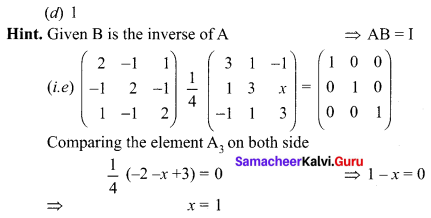Question 25.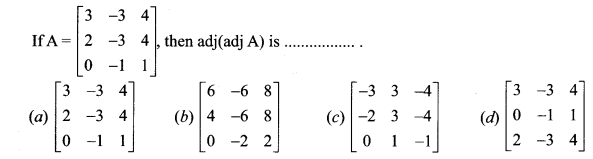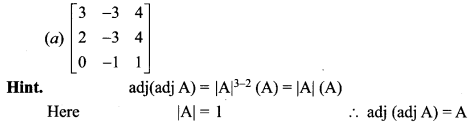### Samacheer Kalvi 12th Maths Solutions Chapter 1 Applications of Matrices and Determinants Ex 1.8 Additional Problems

Question 1.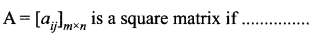(a) m < n (b) m > n
(c) m = n
(d) None of these
Solution:
(c) m = n
Hint:
A = [aij]m×n is a square matrix if number of rows is equal to that of columns, i.e., m = n.

Question 2.
Matrices A and B will be inverse of each other only if ……..
(a) AB = BA
(b) AB = BA = O
(c) AB = O, BA = I
(d) AB = BA = I
Solution:
(d) AB = BA = I
Hint:
By the definition of invertible matrices two matrices A and B are inverse of each other if AB = BA = I.

Question 3.
If A is an invertible matrix of order 2 then det (A-1) is equal to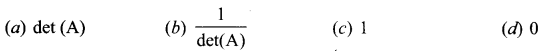Solution: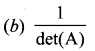Hint.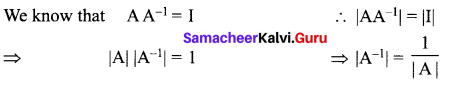Question 4.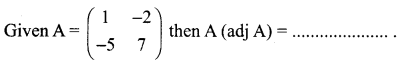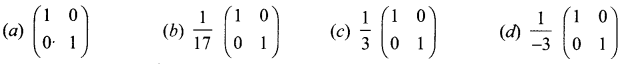Solution:
(d) $$\frac{1}{-3}\left(\begin{array}{ll}{1} & {0} \\ {0} & {1}\end{array}\right)$$

Question 5.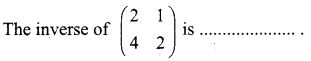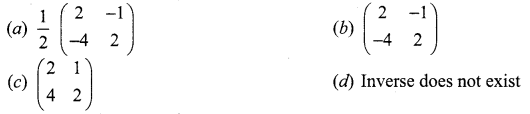Solution:
(d) Inverse does not existQuestion 6.
Given ρ(A, B) = ρ(A) = number of unknowns, then the system has …….
(a) unique solution
(b) no solution
(c) inconsistent
(d) infinitely many solution
Solution:
(a) unique solution

Question 7.
Given ρ(A, B) ≠ ρ(A) then the system has
(a) no solution
(b) unique solution
(c) infinitely many solution
(d) None
Solution:
(a) no solution

Question 8.
Given ρ(A, B) = ρ(A) < number of unknowns, then the system has …….
(a) unique solution
(b) no solution
(c) 3 solutions
(d) infinitely many solution
Solution:
(d) infinitely many solution

Question 9.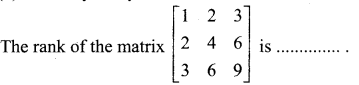(a) 1
(b) 2
(c) 3
(d) None of these
Solution:
(a) 1

Question 10.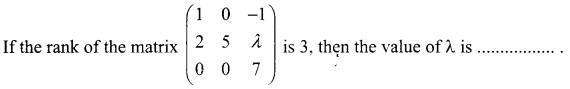(a) 1
(b) 0
(c) 4
(d) any real number
Solution:
(d) any real number

Question 11.
If A = [2 0 1], then the rank of AAT is ………
(a) 1
(b) 2
(c) 3
(d) 0
Solution:
(a) 1
Hint.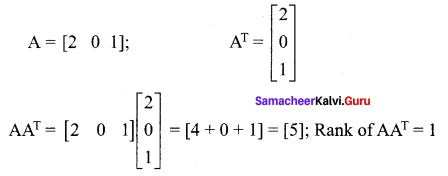Question 12.
If the rank of the matrix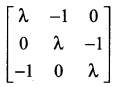is 2, then λ is ……
(a) 1
(b) 2
(c) 3
(d) any real number
Solution:
(a) 1
Hint.
Since the rank of the given matrix is 2, the value of 3rd order determinant is zero.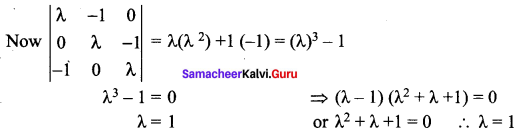Question 13.
If A is a scalar matrix with scalar k ≠ 0, of order 3, then A-1 is …….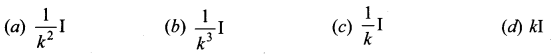Solution:
$$\frac{1}{k} \mathrm{I}$$
Hint: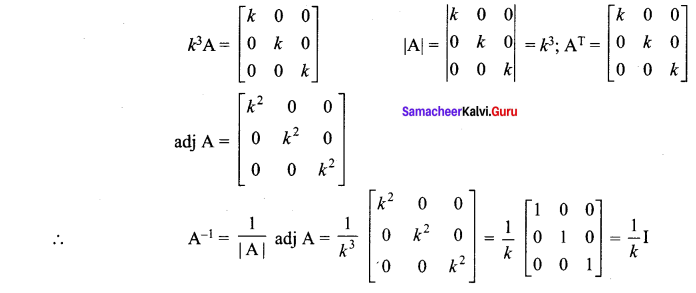Question 14.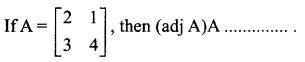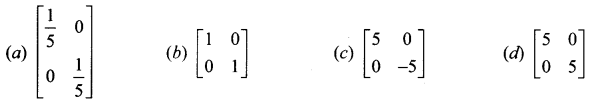Solution:Hint: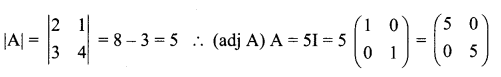Question 15.
If A is a square matrix of order n, then |adj A| is …….
(a) |A|2
(b) |A|n
(c) |A|n – 1
(d) |A|
Solution:
(c) |A|n – 1
Hint:
When A is square matrix of order 3, then |adj A| = |A|2 = |A|3 – 1 ∴ When A is a square matrix of order n, |adj A| = |A|n – 1

Question 16.
If A is a matrix of order 3, then det (kA)
(a) k3(det A)
(b) k2(det A)
(c) k(det A)
(d) det (A)
Solution:
(a) k3(det A)
Hint:
A is a matrix of order 3. ∴ det (kA) = k3 (det A)

Question 17.
If I is the unit matrix of order n, where k ± 0 is a constant, then adj(kI) = …….
(d) kn – 1 (adj I)
Solution:
(d) kn – 1 (adj I)
Hint:
When I is a unit matrix of order 3, then adj (kI) = k2 (adj I)
∴ When I is a unit matrix of order n, then adj (kI) = kn – 1 (adj I)

Question 18.
In a system of 3 linear non-homogeneous equations with three unknowns, if ∆ = 0 and ∆x = 0, ∆y ≠ 0 and ∆z = 0, then the system has …..
(a) unique solution
(b) two solutions
(c) infinitely many solutions
(d) no solution
Solution:
(d) no solution
Hint:
When ∆ = 0 ∆x, ∆z = 0 but ∆y ≠ 0 ⇒ that the system is inconsistent.
∴ There is no solution.